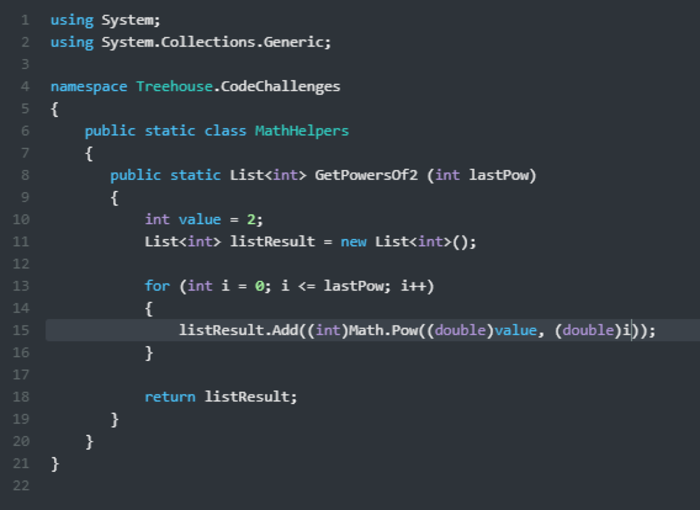can't solve challenge 1 of 1

Challenge Task 1 of 1

Create a static method named GetPowersOf2 that returns a list of powers of 2 from 0 to the value passed in. So if 4 was passed in, the function would return { 1, 2, 4, 8, 16 }. The System.Math.Pow method will come in handy. Bummer! Does MathHelpers.GetPowersOf2 return List`1? Restart Preview Get Help Recheck work MathHelpers.cs

1 using System.Collections.Generic; 2 using System; 3 ​ 4 namespace Treehouse.CodeChallenges 5 { 6 public static class MathHelpers 7 { 8 public static List<double> GetPowersOf2(int input) 9 { 10 var returnList = new List<double>(); 11 for (int i = 0; i < input + 1; i++) 12 { 13 returnList.Add(Math.Pow(2, i)); 14 } 15 return returnList; 16 ​ 17 } 18 ​ 19 } 20 }

MathHelpers.cs
using System.Collections.Generic;
using System;

namespace Treehouse.CodeChallenges
{
public static class MathHelpers
{
public static List<double> GetPowersOf2(int input)
{
var returnList = new List<double>();
for (int i = 0; i < input + 1; i++)
{
}
return returnList;

}

}
}

You forgot to change your two instances of "List<double>" to "List<int>" on lines 8 and 10, the rest is fine.

awesome,thank you so much zack

You're actually very close, I found your problem when trying to figure out my own code challenge and it made me realize what I had forgotten. When you declare both the list for the GetPowersOf2 method, and the returnList, you must declare int for the list type in order for the challenge to pass. However, Math.Pow only accepts the type double, so you have to use type casting in order to change the Math.Pow return type to int

In short, in addition to changing your two double declarations, all you need to add is the green int below :

Hope this helps, good luck!

thank you zack ,but still not working, Challenge Task 1 of 1

Create a static method named GetPowersOf2 that returns a list of powers of 2 from 0 to the value passed in. For example, if 4 is passed in, the method should return this list of integers: { 1, 2, 4, 8, 16 }. The System.Math.Pow method will come in handy. Bummer! Does MathHelpers.GetPowersOf2 return List`1? Restart Preview Get Help Recheck work MathHelpers.cs

1 using System.Collections.Generic; 2 using System; 3 ​ 4 namespace Treehouse.CodeChallenges 5 { 6 public static class MathHelpers 7 { 8 public static List<double> GetPowersOf2(int input) 9 { 10 var returnList = new List<double>(); 11 for (int i = 0; i < input + 1; i++) 12 { 13 returnList.Add((int)Math.Pow(2, i)); 14 } 15 return returnList; 16 ​ 17 } 18 ​ 19 } 20 }using System;
using System.Collections.Generic;

namespace Treehouse.CodeChallenges
{
public static class MathHelpers
{
public static List <int> GetPowersOf2 (int UpperLimit)
{
Console.WriteLine ("What is the upper limit of the Powers of 2 list you request?");

var PowersOf2List = new List <int> ();

for (int index = 0; index < UpperLimit + 1; index++)
{
PowersOf2List.Add ( (int) Math.Pow (2, index) );
}
return PowersOf2List;
}
}
}

very close, try changing List<double> to List<int> then change returnList to....The challenge is apparently expecting a list of integers to be returned.

They probably should have mentioned that. You might want to report it as a bug on the Support page.PRO

My answer to the Challenge

using System;
using System.Collections.Generic;

namespace Treehouse.CodeChallenges
{
public static class MathHelpers
{
public static List<int> GetPowersOf2(int num)
{
int listSizes = num + 1;
double list;
List<int> math = new List<int>(listSizes);

for (int i = 0; i < math.Capacity; i++)
{
list = Math.Pow(2, i);
}

return math;
}
}
}using System.Collections.Generic;
using System;
namespace Treehouse.CodeChallenges
{
public static class MathHelpers
{
public static List<int> GetPowersOf2 (int value)
{
List<int> returnList = new List<int>(value+1);
for (int i = 0 ; i < returnList.Capacity; i++)
{